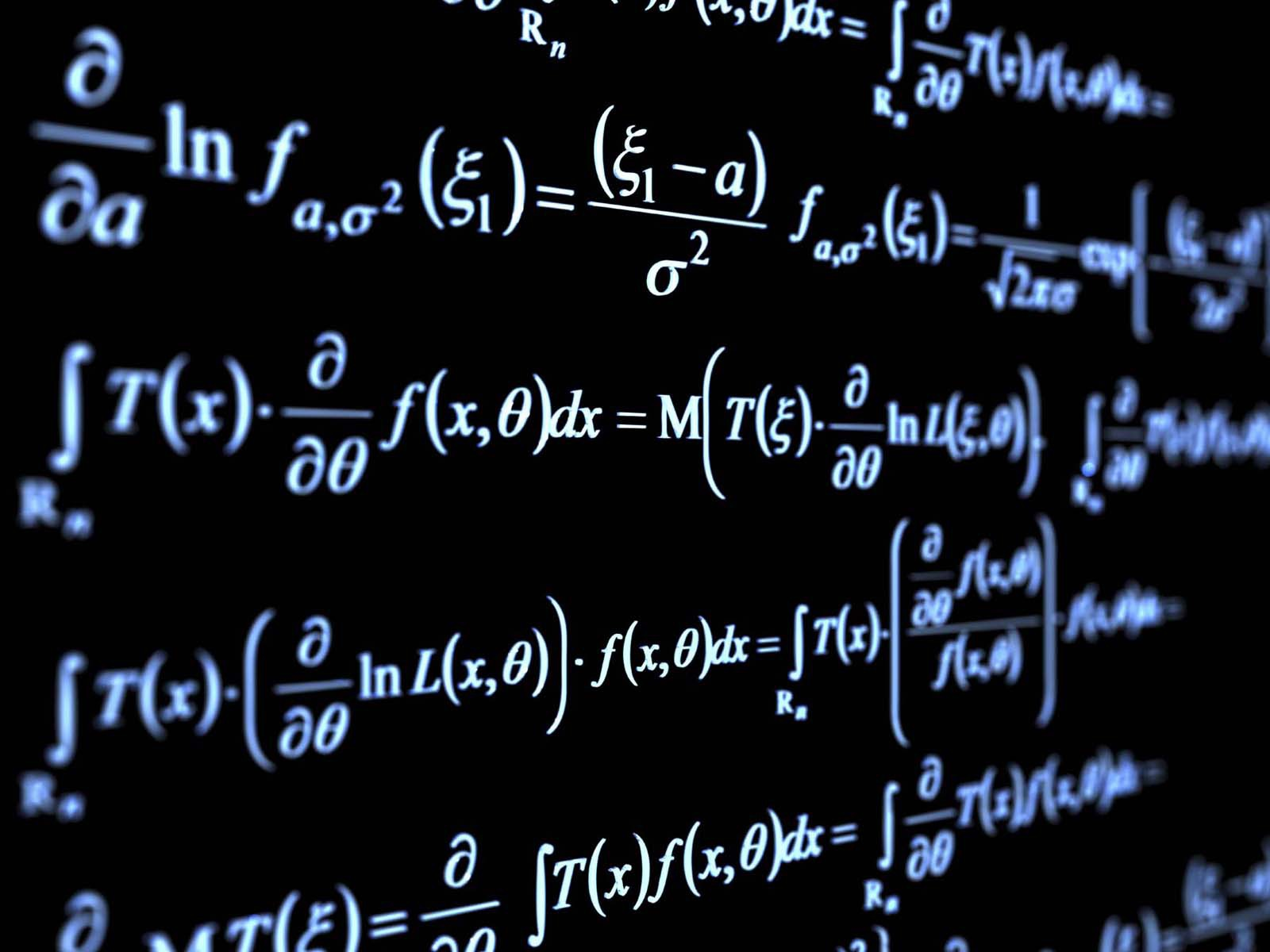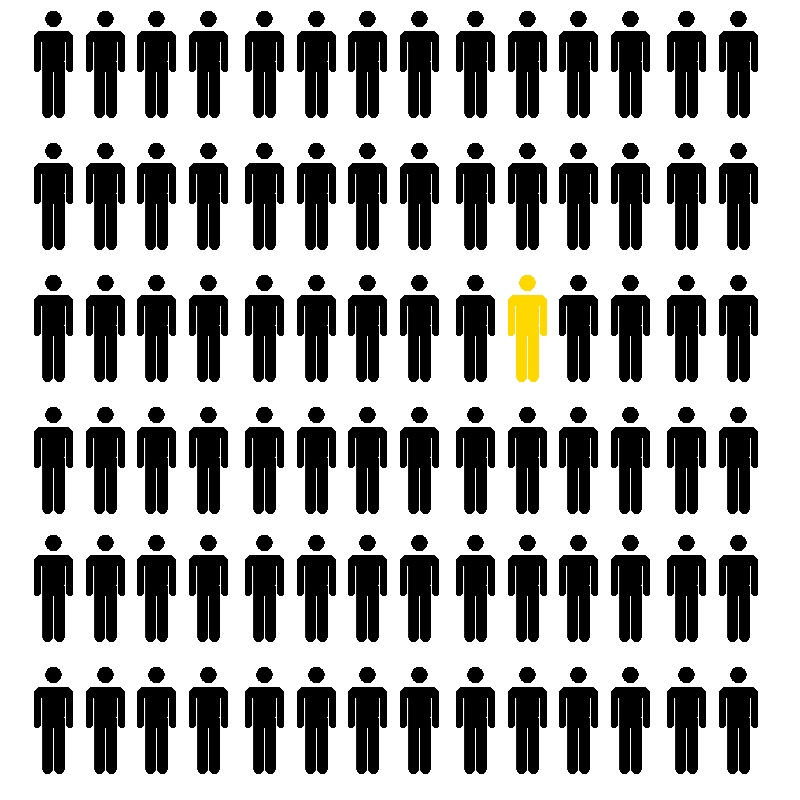Computer Simulations

How do Computer Simulations work?

When a computer simulation is being run, first you need to set up a sequence of mathematical formulas to predict/simulate the outcome. Then, you need to set the input specifications for the simulation to run(e.g. Heat, speed, wind, etc.). These conditions can be changed to more accurately model real-world conditions. TLDR: When the simulation is run, the computer takes the input, uses that information, runs it through special mathematical formulas, and gives an output.Nearly every single simulation run is unique in some way or another, and because of that, every single formula will be slightly different, so there isn’t just one line that you can slap in anywhere and expect it to work. As one would expect, input sources also vary widely. A few examples include sensors and other physical devices connected to the model, Current or historical data entered by hand, values extracted as a by-product from other processes, etc.

As an example of a situation, if someone wanted to simulate how the wind would blow some paper, they would first need to make a formula/use an already existing one that would simulate the reaction. In order to then make use of the formula, that person would then need to define a few parameters such as the weight of the paper, the wind speed, even the air resistance if need be. After which, the simulation would be run, and then the program should simulate the results and would likely display an output.Next Page